# R语言实现评估随机森林模型以及重要预测变量的显著性

08/24 17:01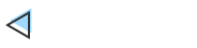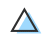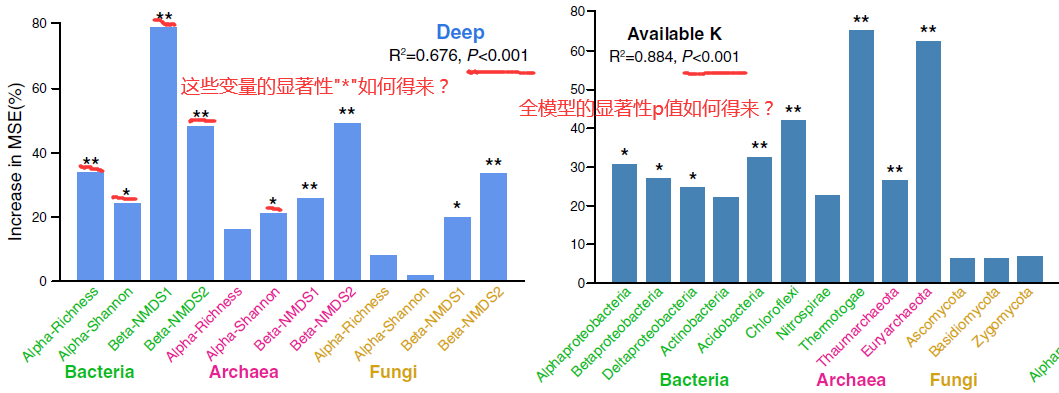randomForest包实现不了的功能，那就用其它R包进行补充呗。至于用哪些R包可以，文献中通常都有详细的方法描述，仔细看一下材料方法部分大致就明确了。就以上面的Jiao等（2018）的文章为例，材料方法部分提到可通过A3包可获取对全模型显著性估计，并可通过rfPermute包可获取对随机森林中预测变量重要性的显著水平估计。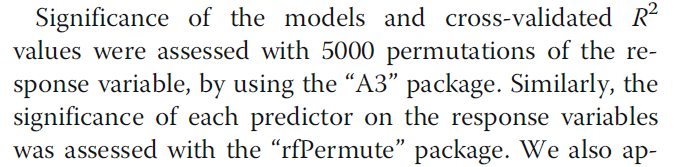https://pan.baidu.com/s/1-L78HuRzZCvH2LCzys4wJQ

https://github.com/lyao222lll/sheng-xin-xiao-bai-yu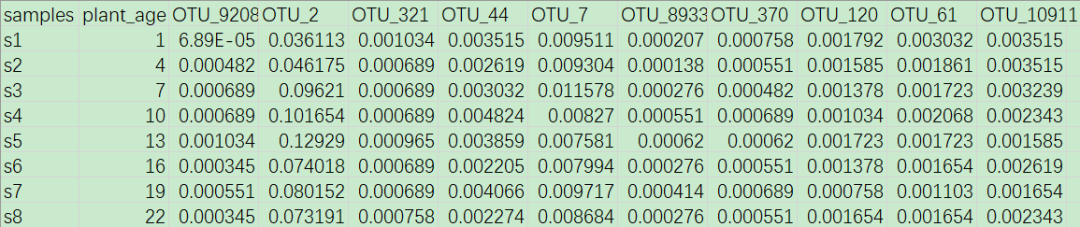#读取 OTU 丰度表#包含预先选择好的 10 个重要的 OTU 相对丰度以及这 45 个根际土壤样本对应的植物生长时间（天）otu <- read.delim('otu_top10.txt', row.names = 1) ##randomForest 包的随机森林library(randomForest) #随机森林计算（默认生成 500 棵树），详情 ?randomForestset.seed(123)otu_forest <- randomForest(plant_age~., data = otu, importance = TRUE, ntree = 500)otu_forest #使用函数 importance() 查看表示每个预测变量（细菌 OTU）重要性的得分（标准化后的得分）importance_otu.scale <- data.frame(importance(otu_forest, scale = TRUE), check.names = FALSE)importance_otu.scale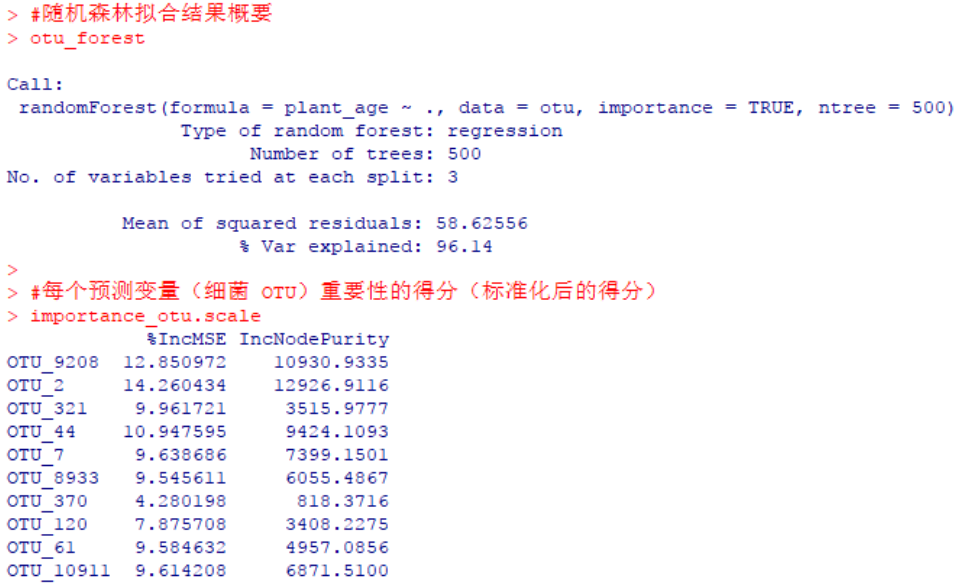#对预测变量（细菌 OTU）按重要性得分排个序，例如根据“%IncMSE”importance_otu.scale <- importance_otu.scale[order(importance_otu.scale$'%IncMSE', decreasing = TRUE), ] #简单地作图展示预测变量（细菌 OTU）的 %IncMSE 值library(ggplot2) importance_otu.scale$OTU_name <- rownames(importance_otu.scale)importance_otu.scale$OTU_name <- factor(importance_otu.scale$OTU_name, levels = importance_otu.scale$OTU_name) p <- ggplot(importance_otu.scale, aes(OTU_name, %IncMSE)) +geom_col(width = 0.5, fill = '#FFC068', color = NA) +labs(title = NULL, x = NULL, y = 'Increase in MSE (%)', fill = NULL) +theme(panel.grid = element_blank(), panel.background = element_blank(), axis.line = element_line(colour = 'black')) +theme(axis.text.x = element_text(angle = 45, hjust = 1)) +scale_y_continuous(expand = c(0, 0), limit = c(0, 16)) p #右上角备注模型的已知解释率p <- p +annotate('text', label = 'Plant Age', x = 9, y = 15, size = 4) +annotate('text', label = sprintf('italic(R^2) == %.2f', 96.14), x = 9, y = 13, size = 3, parse = TRUE) p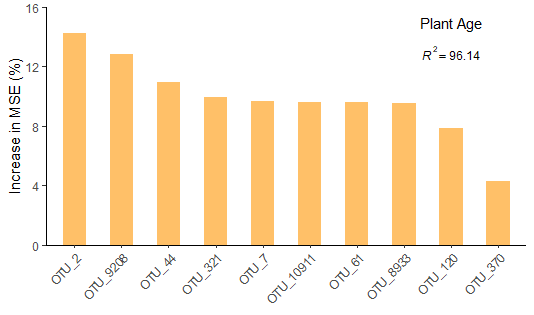通过rfPermute包执行随机森林回归以及获取变量的显著性 尽管上文randomForest包通过计算预测变量的相对重要性得分，允许我们根据得分排名从中确定预测变量的可靠程度，但没有告知我们这些变量是否是显著的。毕竟有些情况下我们确实想迫切知道变量的显著性，如Jiao等（2018）里的那样（本文开篇截图所示文献），因为这些统计量在这些情况中可能很有用。但由于randomForest()函数没有提供估计p值的方法（虽然它有个参数nPerm，但很可惜并不是计算p值的功能），就会很困扰。 仿照Jiao等（2018）的方法，我们可以使用rfPermute包的随机森林去评估每个预测变量（用于回归的10个细菌OTU）对响应变量（植物年龄）的重要性，并获得显著性信息。其实在使用过程中不难看出，rfPermute包沿用了randomForest包的随机森林方法，并对randomForest包的功能作了一些拓展。事实上，我们其实可以跳过randomForest包，直接通过rfPermute包对上文给定的数据执行随机森林分析，会得到和randomForest包一样的运行结果。然后rfPermute包的优势在于给出预测变量重要性得分的同时，还基于置换检验的原理对重要性得分进行了检验，并提供了显著性信息。 library(rfPermute)#使用函数 rfPermut() 重新对上述数据执行随机森林分析，详情 ?rfPermut#rfPermut() 封装了 randomForest() 的方法，因此在给定数据和运行参数一致的情况下，两个函数结果也是一致的#并在这里额外通过 nrep 参数执行 1000 次的随机置换以评估变量显著性的 p 值#若数据量较大，可通过 num.cores 参数设置多线程运算set.seed(123)otu_rfP <- rfPermute(plant_age~., data = otu, importance = TRUE, ntree = 500, nrep = 1000, num.cores = 1)otu_rfP #提取预测变量（细菌 OTU）的重要性得分（标准化后的得分）importance_otu.scale <- data.frame(importance(otu_rfP, scale = TRUE), check.names = FALSE)importance_otu.scale #提取预测变量（细菌 OTU）的重要性得分的显著性（以标准化后的得分为例）# summary(otu_rfP)importance_otu.scale.pval <- (otu_rfP$pval)[ , , 2]importance_otu.scale.pval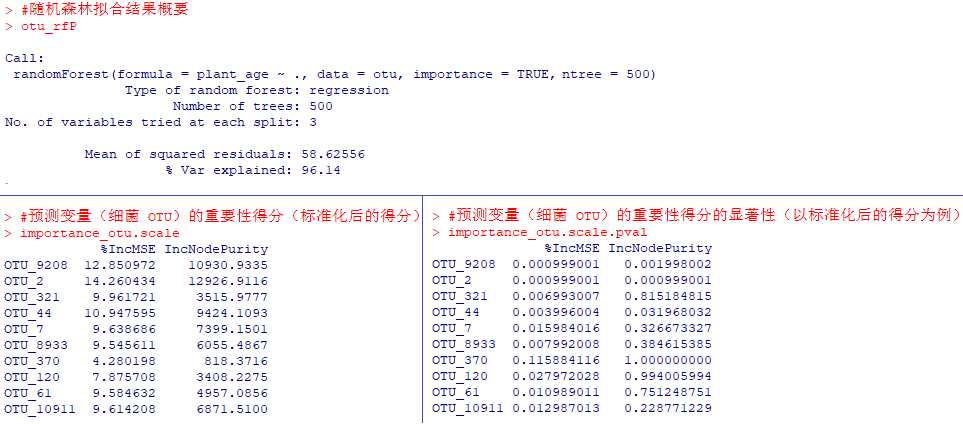#作图展示预测变量（细菌 OTU）的重要性得分（标准化后的得分），其中显著的得分（默认 p<0.05）以红色显示plot(rp.importance(otu_rfP, scale = TRUE))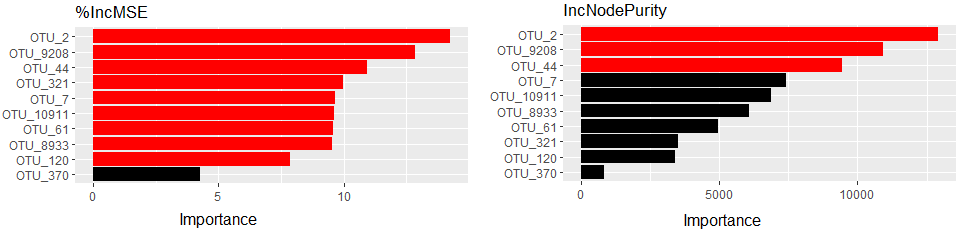#对预测变量（细菌 OTU）按重要性得分排个序，例如根据“%IncMSE”importance_otu.scale <- importance_otu.scale[order(importance_otu.scale$'%IncMSE', decreasing = TRUE), ] #简单地作图展示预测变量（细菌 OTU）的 %IncMSE 值library(ggplot2) importance_otu.scale$OTU_name <- rownames(importance_otu.scale)importance_otu.scale$OTU_name <- factor(importance_otu.scale$OTU_name, levels = importance_otu.scale\$OTU_name) p <- ggplot() +geom_col(data = importance_otu.scale, aes(x = OTU_name, y = %IncMSE), width = 0.5, fill = '#FFC068', color = NA) +labs(title = NULL, x = NULL, y = 'Increase in MSE (%)', fill = NULL) +theme(panel.grid = element_blank(), panel.background = element_blank(), axis.line = element_line(colour = 'black')) +theme(axis.text.x = element_text(angle = 45, hjust = 1)) +scale_y_continuous(expand = c(0, 0), limit = c(0, 16)) p #标记预测变量（细菌 OTU）的显著性信息#默认以 p<0.05 为 *，p<0.01 为 **，p<0.001 为 ***for (OTU in rownames(importance_otu.scale)) {    importance_otu.scale[OTU,'%IncMSE.pval'] <- importance_otu.scale.pval[OTU,'%IncMSE']    if (importance_otu.scale[OTU,'%IncMSE.pval'] >= 0.05) importance_otu.scale[OTU,'%IncMSE.sig'] <- ''    else if (importance_otu.scale[OTU,'%IncMSE.pval'] >= 0.01 & importance_otu.scale[OTU,'%IncMSE.pval'] < 0.05) importance_otu.scale[OTU,'%IncMSE.sig'] <- '*'    else if (importance_otu.scale[OTU,'%IncMSE.pval'] >= 0.001 & importance_otu.scale[OTU,'%IncMSE.pval'] < 0.01) importance_otu.scale[OTU,'%IncMSE.sig'] <- '**'    else if (importance_otu.scale[OTU,'%IncMSE.pval'] < 0.001) importance_otu.scale[OTU,'%IncMSE.sig'] <- '***'} p <- p +geom_text(data = importance_otu.scale, aes(x = OTU_name, y = %IncMSE, label = %IncMSE.sig), nudge_y = 1) p #右上角备注模型的已知解释率p <- p +annotate('text', label = 'Plant Age', x = 9, y = 15, size = 4) +annotate('text', label = sprintf('italic(R^2) == %.2f', 96.14), x = 9, y = 13, size = 3, parse = TRUE) p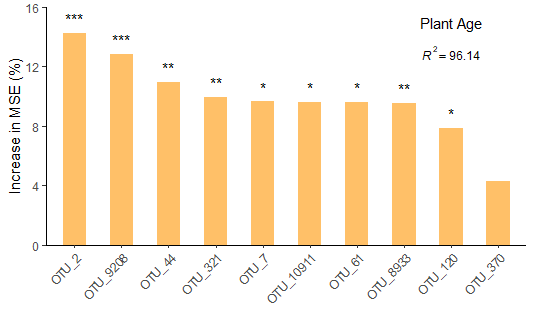library(A3)#model.fn=randomForest 调用随机森林的方法进行运算#p.acc=0.001 表示基于 1000 次随机置换获得对 p 值的估计，p.acc 值越小代表置换次数越多，运算也就越慢，因此如果对全模型 p 值不是很迫切的话还是慎用#model.args 用于传递参数给 randomForest()，因此里面的参数项根据 randomForest() 的参数项而定，具体可 ?randomForest#其它详情可 ?a3 查看帮助 set.seed(123)otu_forest.pval <- a3(plant_age~., data = otu, model.fn = randomForest, p.acc = 0.001, model.args = list(importance = TRUE, ntree = 500))otu_forest.pval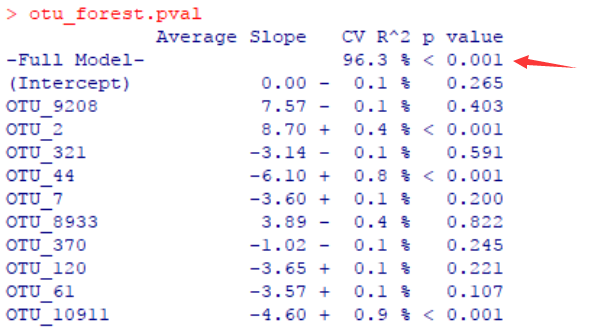#继续在右上角备注全模型的显著性信息p <- p +annotate('text', label = sprintf('italic(P) < %.3f', 0.001), x = 9, y = 12, size = 3, parse = TRUE) p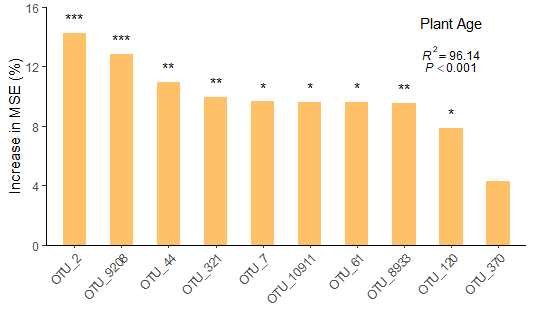Jiao S, Chen W, Wang J, et al. Soil microbiomes with distinct assemblies through vertical soil profiles drive the cycling of multiple nutrients in reforested ecosystems. Microbiome, 2018, 6(1): 1-13.

rfPermute包：https://www.rdocumentation.org/packages/rfPermute/versions/2.1.81

A3包：https://rdrr.io/cran/A3/Aggregated boosted treeABT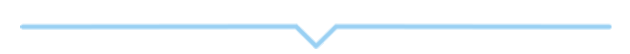0
0 收藏

### 作者的其它热门文章0 评论
0 收藏
0